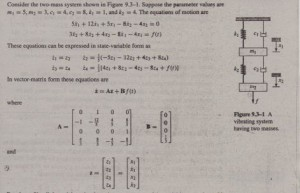Develop a Simulink model to plot the unit-step response of the variables XI and X2 with the initial conditions XI (0) = 0.2, XI (0) = 0, X2(0) = 0.5, X2(0) = 0.
Solution
First select appropriate values for the matrices in the output equation y = Cz +Bf(t). Since we want to plot XI and X2, which are Zl and Z2, we choose c and D as follows.
C = [I 0 0 0] D 
0010 =0
To create this simulation, obtain a new model window. Then do the following to create the model shown in Figure 9.3-2.
1. Select and place in the new window the Step block. Double-click on it to obtain the Block Parameters window, and set the Step time to 0, the Initial and Final values to 0 and I, and the Sample time to O. Click OK. The Step time is the time at which the step input begins.

2. Select and place the State-Space block. Double-click on it, and enter [0, 1, 0,
0; -1, -12/5, 4/5, 8/5; 0, 0, 0, 1; 4/3, 8/3, -4/3, -8/3]
for A, [0; 0; 0; 1/3] for 8, [1, 0, 0, 0; 0, 0, 1, 0] for C, and
[0; 0] for D. Then enter [0.2; 0; O. 5; 0] for the initial conditions. Click OK. Note that the dimension of the matrix 8 tells Simulink that there is one input. The dimensions of the matrices C and D tell Simulink that there are two outputs.
3. Select and place the Scope block.
4. Once the blocks have been placed, connect the input port on each block to the output port on the preceding block as shown in the figure.
5. Experiment with different values of the Stop time until the Scope shows that the steady-state response has.been reached. For this application, a Stop time of 25 is satisfactory. The plots of both XI and X2 will appear in the Scope